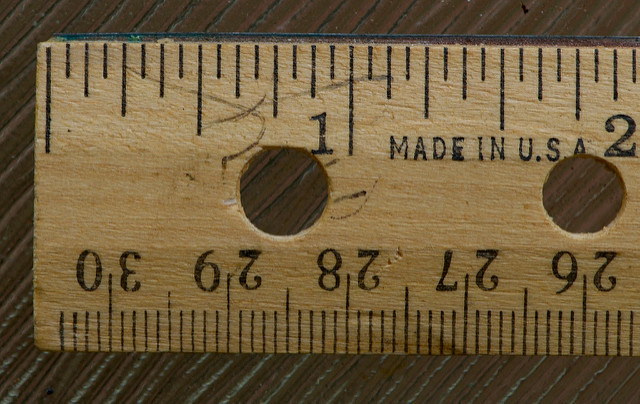# Reviewing Conversion Factors# Explore Centimeters and Inches

• Open the interactive and move the slider above the ruler to 1 inch.
About how many centimeters are equal to 1 inch?

• Move the slider below the ruler to 1 centimeter.
About how many inches are equal to 1 centimeter?

INTERACTIVE: Interactive Ruler

## Hint:

Use the sliders on the ruler to find rates.

1 of 8